爱丽丝的发丝──《爱丽丝惊魂记：疯狂再临》制作点滴

2011-06-14 16:27  Milo Yip  阅读(99082)  评论(110编辑  收藏  举报

简介

《爱》(图1a)是一款由上海独立游戏工作室麻辣马(Spicy Horse)制作、美商电艺(Electronic Arts)发行的惊悚动作冒险游戏。此全乃2000年发行的《爱丽丝惊魂记(American McGee’s Alice) PC》(图1b)的续作。《爱》使用Unreal Engine 3开发，并使用了ScaleformKynapseBink中间件。在PC平台上，合作伙伴nVidia加入了使用GPU加速PhysX效果。但游戏主角爱丽丝的头发和衣饰模拟，并非使用PhysX，而是一个自定义解决方案，这也是本文将谈及的主要内容。

研究之始

2009年8月23日(星期日)，刚入职满三个星期了。这段时间的工作，主要是按游戏策画的需求，做了一些简单的游戏性编程(gameplay programming)，例如是一些关卡内的机关，这正好让我学习一下UnrealScript(Unreal引擎的脚本语言)。

弹簧质点系统\mathbf{x}(t + \Delta t) = \mathbf{x}(t) + d \cdot (\mathbf{x}(t) - \mathbf{x}(t - \Delta t)) + \mathbf{a}(t) \Delta t^2

Verlet积分的另一特点，是可以简单地加入各种约束(constraint)，例如某粒子在仿真之后，其位置位于地面以下，只需把粒子移至最近地面的点。对于绳子，另一约束就是相邻粒子的距离，在Verlet积分下，此距离约束可以模拟弹簧。假设两个相邻粒子的位置为x_1x_2，两者间的止动长度(rest length)为L_r，则可以这样调节两粒子的位置：

\begin{matrix} \mathbf{x}’_1 = \mathbf{x}_1 + (\mathbf{x}_2 - \mathbf{x}_1) \cdot \frac{\left \| \mathbf{x}_2 - \mathbf{x}_1 \right \| - l_r}{2 \left \| \mathbf{x}_2 - \mathbf{x}_1 \right \|} \\ \mathbf{x}’_2 = \mathbf{x}_2 - (\mathbf{x}_2 - \mathbf{x}_1) \cdot \frac{\left \| \mathbf{x}_2 - \mathbf{x}_1 \right \| - l_r}{2 \left \| \mathbf{x}_2 - \mathbf{x}_1 \right \|} \end{matrix}

// 节点(粒子)
struct Node {
Vector3 p0, p1; // 前帧/本帧的位置
float length; // 和上一节点的止动长度
}

// 发束
struct Strand {
size_t nodeStart, nodeEnd; // 此发束中，节点数组的起始和结束索引
Vector3 rootP; // 发根的局部坐标(相对于头的变换)
};

SimulateHair(nodes, strands, sphere, damping, dt, headToWorld)
// 对每个节点进行Verlet积分
for each n in nodes
a = Accumulating force for n, divided by mass // 现时只是引力加速度常量
p2 = Verlet(n.p0, n.p1, damping, a, dt)
n.p0 = n.p1, n.p1 = p2 // 以新状态取代旧状态

// 对每束发丝以松弛法进行约束求解
for each s in strands
for a number of iterations
for index = s.nodeStart to s.nodeEnd - 1
na = nodes[index]
nb = nodes[index + 1]
// 碰撞检测和决议
nb.p1 = collideSphere(sphere, nb.p1)
// 长度约束
na.p1, nb.p1 = lengthConstraint(na.p1, nb.p1, nb.length)

// 固定发根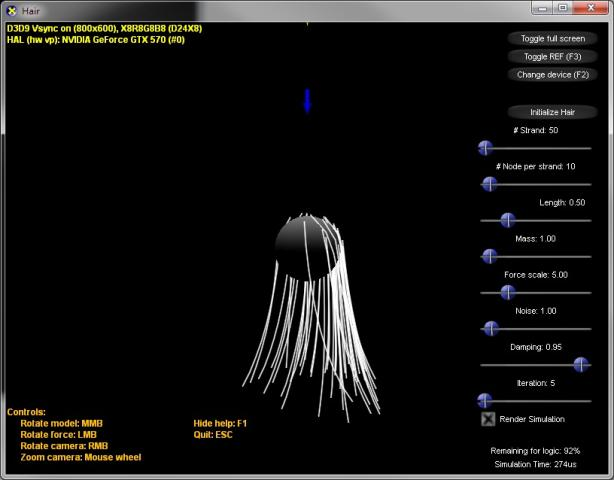头发渲染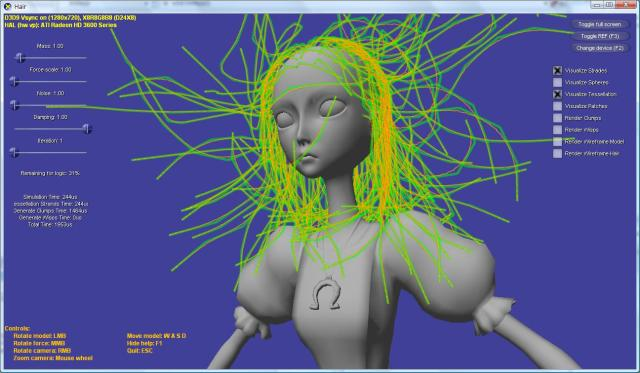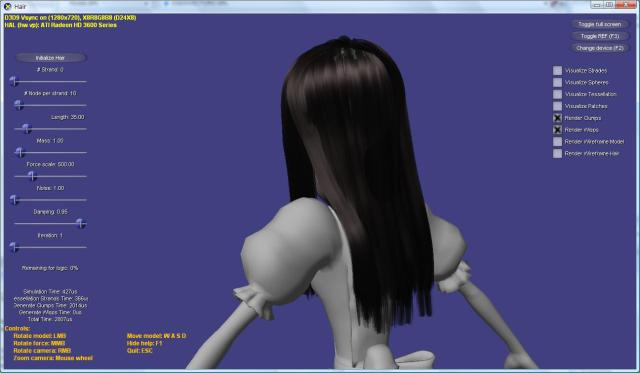改进模拟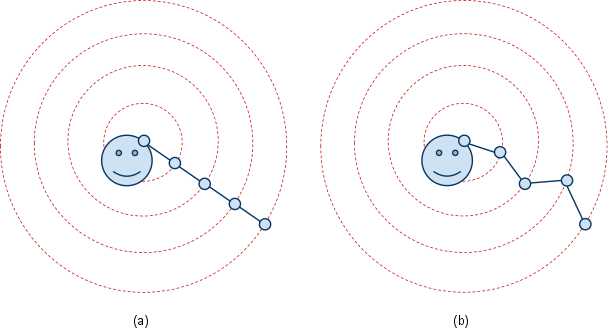效能测试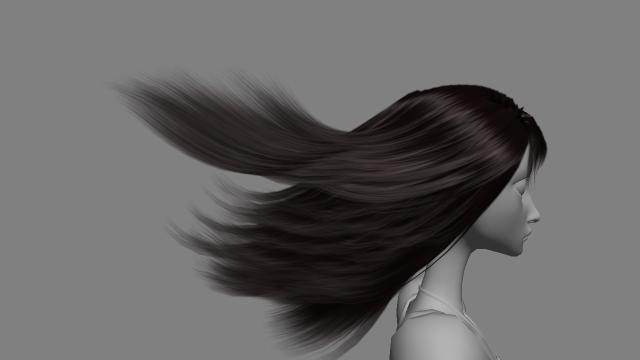新的需求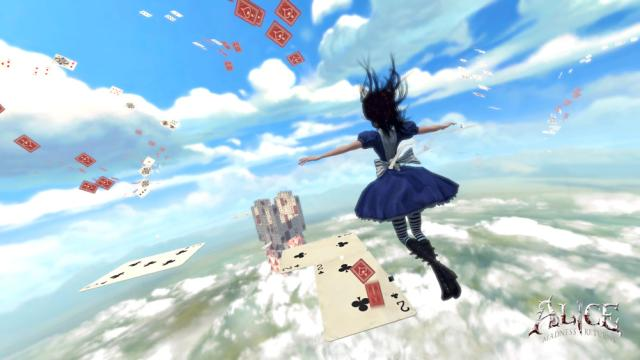结语